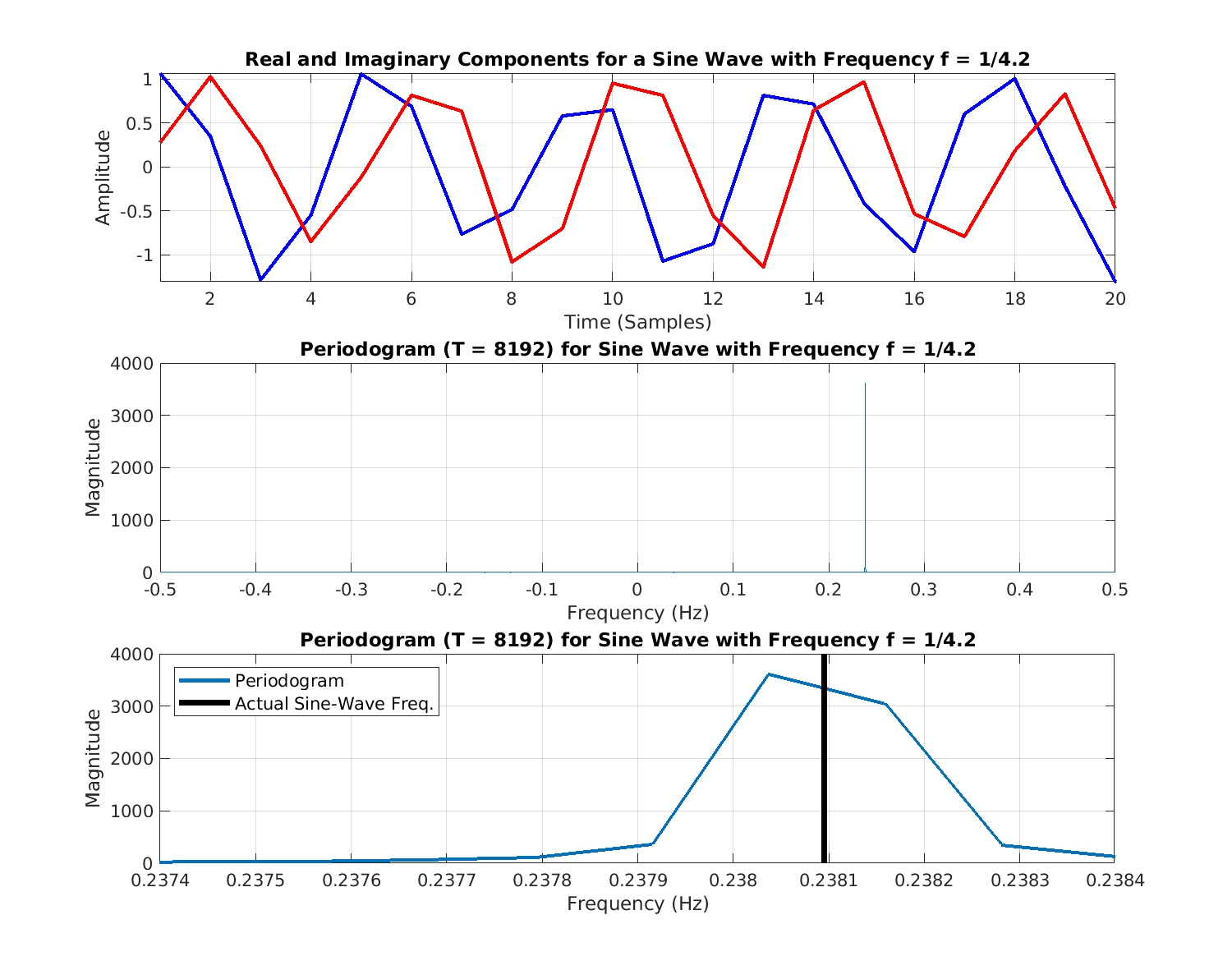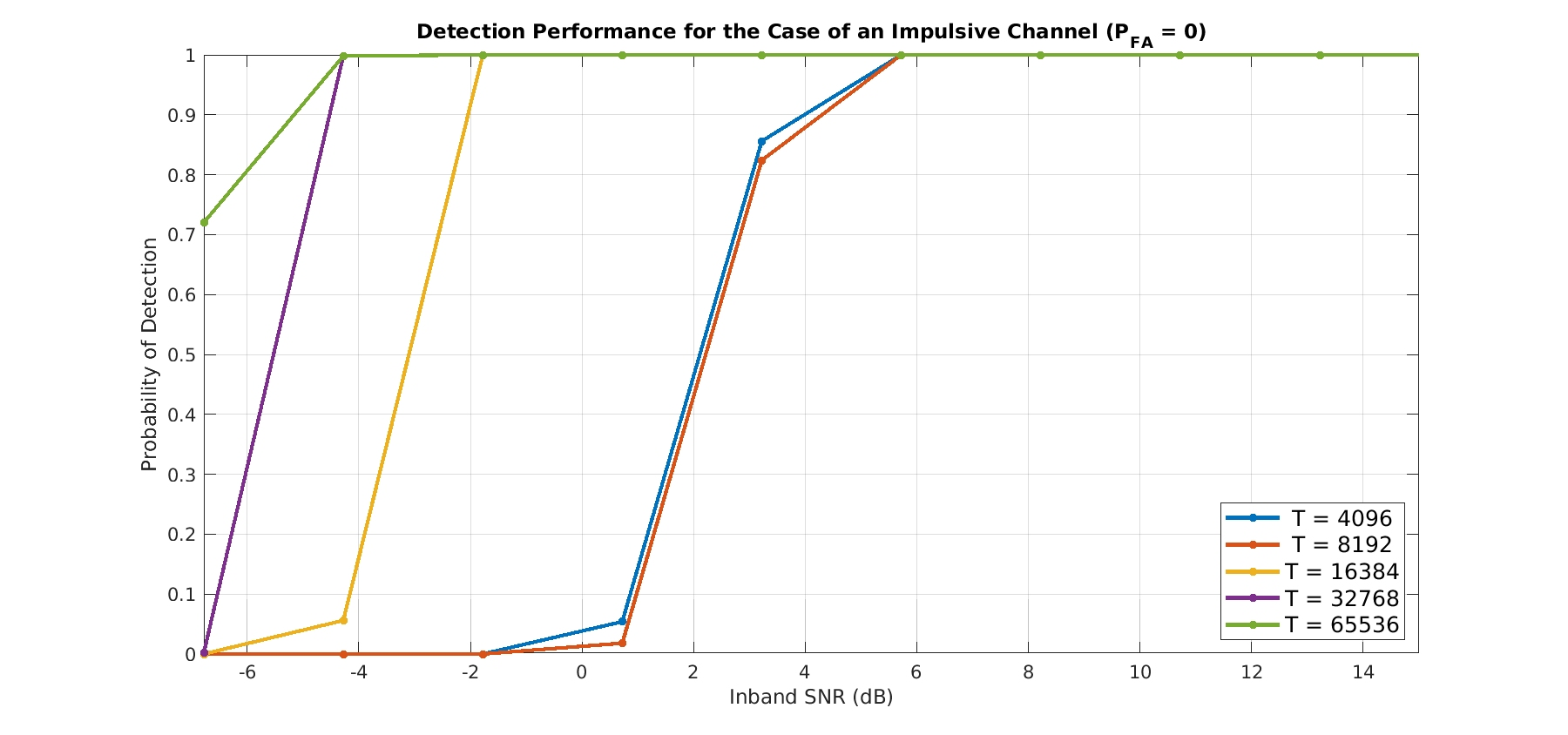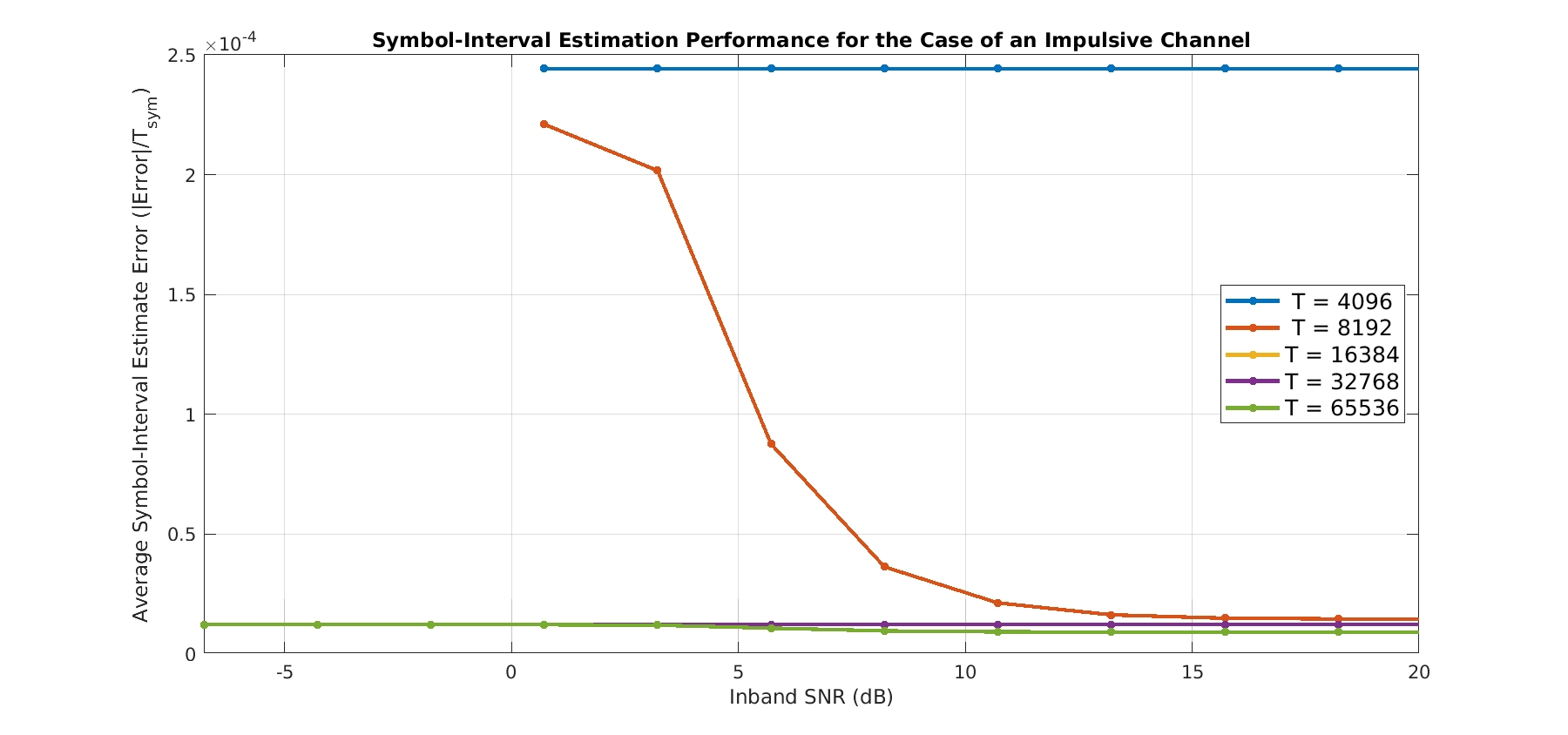# Comments on “Detection of Almost-Cyclostationarity: An Approach Based on a Multiple Hypothesis Test” by S. Horstmann et al

The statistics-oriented wing of electrical engineering is perpetually dazzled by [insert Revered Person]’s Theorem at the expense of, well, actual engineering.

I recently came across the conference paper in the post title (The Literature [R101]). Let’s take a look.

The paper is concerned with “detect[ing] the presence of ACS signals with unknown cycle period.” In other words, blind cyclostationary-signal detection and cycle-frequency estimation. Of particular importance to the authors is the case in which the “period of cyclostationarity” is not equal to an integer number of samples. They seem to think this is a new and difficult problem. By my lights, it isn’t. But maybe I’m missing something. Let me know in the Comments.

The set-up is the reception of a QPSK signal with square-root raised-cosine pulses and a symbol rate of$f_{sym} = 1/4.2 \approx 0.23809523809523809523.$ The problem, as they see it, is that$0.2$ part of the$4.2$. They cite a couple of CSP detectors that use prior knowledge of the cycle frequency (like the cycle detectors do) and some algorithms that produce estimates of the cycle frequency provided it is equal to an integer number of samples. Since they don’t have prior knowledge, and they don’t want to constrain their cycle frequency to the reciprocal of an integer, these algorithms and approaches are dismissed.

However, they don’t mention or cite the long-standing blind cycle-frequency estimation methods published by Gardner, Brown, Loomis, and Roberts (The Literature [R3, R4] ). I’m talking about, of course, algorithms like the strip spectral correlation analyzer and the FFT accumulation method. There are simpler methods as well, such as forming the second-order lag product with one conjugated factor and then performing conventional Fourier-based sine-wave-frequency estimation.

To cut to the chase, recall that if we process a data block having length$T$ samples, the cycle frequencies exhibited by the signals in the data can be estimated to an accuracy of approximately 1/T. Algorithms like the SSCA and FAM produce cycle-frequency estimates having the form$k/T$, and simple interpolation or binning post-processing can improve the accuracy by using weighted averages involving closely spaced cycle-frequency estimates for a variety of spectral frequencies. So, suppose we process with$T = 8192$ samples. Then cycle frequencies are estimated with errors no larger than about$1/T = 0.00122$ Hz (normalized frequencies are used throughout this post), and so the endpoints of the range of cycle-frequency estimates are given by$\displaystyle 1/4.2 \pm 1/T = \{0.237973, 0.2382173\}. \hfill (1)$

These two errors imply estimates of the symbol interval of$\{4.19785, 4.20215\}$, or percentage errors of no worse than$100 \times \frac{0.00215}{4.2} = 0.5\%$.

Moreover, for detection, the coherence can be used together with the SSCA or FAM to  determine the set of statistically significant cycle frequencies. Because the resolution of the cycle-frequency estimates is approximately$1/T$, any cycle frequency whatsoever can be detected using these methods—even irrational ones. Using our digital signal processing in this manner, we will never produce an irrational estimate, but if the cycle frequency is exactly irrational, we will simply produce an estimate that is close. How close? Well, to within$1/T$ Hz.

### Connection to Sine-Wave Frequency Estimation

The authors of The Literature [R101] don’t seem to use any knowledge of the frequency-domain parts of cyclostationary signal processing. It’s as if they were confronted with a sine-wave frequency estimation problem where the number of samples per period is not an integer, and then decided that the periodogram just doesn’t apply, and that processing has to take place in the time domain. But the periodogram does still apply.

This really is the crux of the matter. The periodogram can be used to find an estimate of the frequency (and amplitude and phase if desired) of a sine wave in noise (or even in interference). When using discrete time and discrete frequency, we end up computing the DFT via the FFT. When processing a data block having$T$ samples, the FFT output has$T$ frequencies, which are commonly referred to as bins. Energy in the input signal that lies close to a bin gets mostly reflected by that bin’s FFT magnitude. There is no restriction on the nature of the sine-wave frequency in the input data block. If it lies halfway between two bins (between two FFT output frequencies), about half of the energy will appear in one bin, and the other half in an adjacent bin. Some of the energy is reflected in the other bins too, just not very much.

If we simulate a sine wave with frequency$f_0 = 1/4.2 \approx 0.23809523809523809523$, we can use the periodogram to find an estimate of the frequency and therefore an estimate of the period:Figure 1. Periodogram for a noiseless sine wave with a frequency of exactly 1/4.2 Hz. The periodogram is computed for frequencies k/T = k/8192, and the sine-wave frequency falls between two such frequencies. So about half the energy of the sine wave is reflected in each of those two elements of the periodogram (lower plot).

For$T = 8192$, the periodogram has its peak at$f = 0.238037109375$, which corresponds to a period estimate of$4.2010256$. The sine-wave frequency is between two bins for this$T$. For$T = 16384$, however, the sine-wave frequency is very close to a periodogram bin:Figure 2. Periodogram for a noiseless sine wave with a frequency of exactly 1/4.2 Hz. The periodogram is computed for frequencies k/T = k/16384, and the sine-wave frequency is very nearly equal to one of those visited frequencies. So nearly all the sine-wave energy is captured by a single periodogram value (lower plot).

In this case, the period estimate is$4.1999487$.

Let’s move on to SSCA-based simulations using the parameters in the paper.

### SSCA Simulation Results

To create the QPSK signal with a symbol interval of$4.2$, I first created one with a symbol interval of$21$ and then decimated it by a factor of$5$. So the frequency is exactly what we want, not approximately what we want. I considered two channels: an impulsive channel (perfect propagation) and the channel used by the authors of The Literature [R101], which has$10$ taps and a particular power-delay profile. To ensure I was using the same channel as the authors, I used the link in the paper to access and download the MATLAB code they used to create their results. The link is https://github.com/SSTGroup/Cyclostationary-Signal-Processing. I then extracted their propagation-channel set up and wrote a MATLAB function that created$1000$ channels, which I stored in a file for use during my Monte Carlo simulations.

One difference between my simulations and the paper’s is that I’m doing single-sensor processing and they use an array (typically with$L= 2$ elements). For each element, they create “M i.i.d. realizations of u[n] of length N”. If I’m following correctly, the total number of samples processed to produce a result is$LMN$.  In some of the results figures, they also use the parameter$S$ for the number of symbols, and leave$N$ unspecified. In such cases, the total number of processed samples is$4.2LMS$, since the true symbol interval is$4.2$ samples. So, for example, for Figure 5, we have$L=2, S = 192, M = 25$, yielding a total number of samples equal to$40320$, or about$20000$ samples per antenna output. So for reasonable comparison with my results, I should take$T$ to be in the low tens of thousands of samples.

I consider$T$ values of$4096, 8192, 16384, 32768,$ and$65536$. Some$T$ are shorter than those used in the paper, some longer, so I think I’ve got the right range.

Regarding SNR, the authors don’t define their SNR measure, but I’m using inband SNR, which is defined as the ratio of the signal power to the noise power in the signal’s occupied bandwidth. (I always use inband SNR at the CSP Blog.) The occupied bandwidth here is$B = 2/4.2 \approx 0.5$, because the QPSK signal has 100 percent excess bandwidth. So if the authors used total SNR, which is defined by the ratio of the signal power to the noise power in the sampling bandwidth, then my measure of SNR is about$3$dB larger than theirs. In other words, my$0$ dB is their$-3$ dB. I end up considering inband SNRs ranging from$-7$ dB to$20$ dB.

For each combination of channel type, inband SNR, and data-block length$T$, I perform$500$ trials on the signal-present hypothesis$H_1$ and$500$ on the signal-absent hypothesis$H_0$. On each trial, I simply generate the data block and apply the SSCA.

In all of the trials on$H_0$, no detected cycle frequencies are observed. That is, the empirical probability of false alarm is zero for my SSCA-based method. Compare with the authors’ choice of$P_{FA} = 0.05$, and their results that show that their empirical (measured)$P_{FA}$ increases with increasing$M$ and increasing$N$.

First, detection performance:Figure 3. Blind detection of the signal’s symbol-rate cycle frequency for the case of a benign additive white Gaussian channel. No cycle frequencies were detected on any trial, meaning the false-alarm probability is zero here.Figure 4. Blind detection of the signal’s symbol-rate cycle frequency for the case of the author’s non-ideal propagation channel. No cycle frequencies were detected on any trial, meaning the false-alarm probability is zero here.

In terms of detection, for$T = 16384$ and$T=32768$, this SSCA method is clearly competitive with the authors’ method (see Figures 5 and 6). In terms of false-alarm performance, this SSCA method is far superior.

Next, symbol-interval estimation performance:Figure 5. Average normalized error in the symbol-interval estimate obtained by inverting the blindly detected cycle frequency obtained using the SSCA.  Here the channel is a benign WGN channel. The average error is a tiny fraction of the symbol interval for the larger processed block lengths, which is a result predicted by the periodogram analysis applied to a sine wave shown in Figures 1 and 2.Figure 6. Average normalized error in the symbol-interval estimate obtained by inverting the blindly detected cycle frequency obtained using the SSCA.  Here the channel is the authors propagation channel. The average error is a tiny fraction of the symbol interval for the larger processed block lengths, which is a result predicted by the periodogram analysis applied to a sine wave shown in Figures 1 and 2.

For either channel, the accuracy of the symbol-interval estimate is very high; the error is a tiny fraction of the true symbol interval of$4.2$ samples.

There is at least one problem with the SSCA-based method. On the signal-present hypothesis$H_1$, the SSCA can produce cycle frequencies that are not associated with the signal. This can happen because the coherence is the primary detection statistic. Recall that the coherence is defined by the ratio$C_x^\alpha(f) = \displaystyle\frac{S_x^\alpha(f)}{[S_x^0(f+\alpha/2)S_x^0(f-\alpha/2)]^{1/2}}. \hfill (2)$

where$S_x^\alpha(f)$ is the spectral correlation function,$S_x^0(f)$ is the power spectral density, and$\alpha$ is the cycle frequency.

When the denominator power spectra are poorly resolved (the power spectra contains narrow fluctuations due to channel distortion, contains additive sine-wave components, or contains spectral features that are like unit-step functions), the quotient can be large (exceeding threshold) even for cycle frequencies that are not exhibited by the signals in the data. We can call these$H_1$ false cycle frequencies.

Here is a summary of the$H_1$ false cycle frequency performance:

The authors of The Literature [R101] don’t consider any symbol interval greater than$10$, which means no cycle frequency less than$0.1$. If we similarly limit our consideration of the blindly estimated cycle frequencies at the output of the SSCA, we obtain:

So for low inband SNRs, this false cycle frequency issue is not a big problem for either channel type. These averages are a little misleading as well, since a small number of trials that happened to produce, say, tens of false cycle frequencies can skew the average. Let’s look at a couple histograms of the number of$H_1$ false cycle frequencies:

You can see that the vast majority of trials on$H_1$ produce a single cycle-frequency estimate (the single correct cycle frequency$1/4.2$). The false-cycle-frequency problem can be mitigated at the expense of decreased probability of detection, but we’ll talk about that another time.

So, in the end, we can apply a decades-old blind cycle-frequency estimation algorithm like the SSCA or FAM and handle the signal that the authors of The Literature [R101] want to handle. Moreover, the false-alarm performance is much better, and we don’t have to resample the signal$85$ times or more to obtain high-accuracy estimates of arbitrary symbol periods—integer or fractional or irrational.

### What’s Going on Here?

This kind of publication is one of the reasons for the existence of the CSP Blog. There are two issues at work here. The first is the general poor state of the review process. It is just too hard to get good reviews, so papers (including conference papers, which are often peer-reviewed) that are flat-out wrong or that ignore relevant previous work can still get published. The second issue relates to the nature of the signal-processing community. Academic researchers are very concerned with optimization problems that can be solved mathematically, and end up presenting and analyzing overly simple versions of problems. Industrial researchers are very concerned with robustness, performance, and processing delay and tend to minimize the importance of a provably optimal solution. The first group generally controls the review process.I'm a signal processing researcher specializing in cyclostationary signal processing (CSP) for communication signals. I hope to use this blog to help others with their cyclo-projects and to learn more about how CSP is being used and extended worldwide.

Posted on

## 5 thoughts on “Comments on “Detection of Almost-Cyclostationarity: An Approach Based on a Multiple Hypothesis Test” by S. Horstmann et al”

1.Jim Costabile says:

Great post as usual. I like forming the lag product and taking the Fourier Transform, or applying other spectral analysis techniques like the chirp z transform to get higher resolution in the area of interest (if you know it apriori, or can estimate it from the observed bandwidth). I also like measuring the effects of raised cos filtering, since as a comms engineer we are always looking to minimize bandwidth and this effects the strength of the cyclostationary feature.

1.Chad Spooner says:

Thank you Jim. Yes, one can get a lot done on practical problems with simple techniques like delay-and-multiply followed by conventional sine-wave estimation. I think a big problem with trying to derive/apply optimal estimators is that the model in the math doesn’t match the data in the field. So you end up with brittle algorithms.

I’ve not used the chirp-Z transform in my algorithm development, but I’m wondering if it might have a role in automatic spectral segmentation. Hmmm.

Yeah, low excess bandwidth QAM/PSK have weak features–this was one of the original motivation factors behind my study of higher-order cyclostationarity.

2.Manish says:

Excellent post Chad. I appreciate your contribution by sharing important technical aspects which is of great help. Right now I am working with Cyclostationarity and Almost-Cyclostationarity aspect of signals. The paper says that sampling a continuous time signal with the sampling period less than the Cyclostationarity period leads to ACS signal in discrete-time. Chad, I disagree with this statement, because what I have found out is that if the sampling period is Tamp and period of cyclostationarity is Tsym, then the discrete -time signal will be CS signal when Tsym/Tsamp=Nysm, where Nsym is a positive integer. On the other hand the discrete-time signal will be ACS signal only when the sampling is non-synchronous, i..e, Tsym/Tsamp=Nsym+e, where e is the asynchronous factor and makes the discrete-time signal ACS instead of CS.

3.Chen says:

1.Chad Spooner says:
Yes, I do not think ACS signals are a problem for the FAM and SSCA. That is the conclusion of the post. In particular, each of those two algorithms provides a set of point estimates of the spectral correlation function on the diamond-shaped spectral correlation principal domain. The resolution of the point estimates in spectral frequency$f$ (called$\Delta f$) and cycle frequency$\alpha$ (called$\Delta\alpha$) is such that all possible cycle frequencies (rational, irrational, lying on the grid of point-estimate centers, not lying on that grid, whatever) can be detected. The corresponding estimate of a cycle frequency will correspond, without further post processing of the set of point estimates, to one of the cycle frequencies in the grid of estimates. In other words, an error-free estimate of a cycle frequency is only possible when the cycle frequency happens to lie on the grid of cycle frequencies implied by the FAM or SSCA estimator structure (that is,$k/N$, where$N$ is the total number of processed samples).